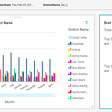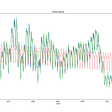# FFT and PSD — normalize values

I have noticed many posts regarding frequency spectrum and many answers to the same questions. How to normalize the values of FFT and PSD from a periodic signal?

Most of us when we refeer to “normalize the frequency domain” we mean that it is required to obtain the frequency domain of the signals where the amplitude of the signal, or the time window, do not affect the obtained plot.

For example in my case I use a periodic sine signal with a frequency of 160HZ, and observed that the amplitude of the foundamental frequency in both figures changes according to the length of the signal. So the questions are:

How to obtain a FFT and PSD where the amplitude values are not affected by:

1. The time window or the number of points.
2. The peak amplitude of the signal. I mean for example if the signal amplitude is reduced by the half, still getting the corresponding peak value, or even better the corresponding percentage.

Notice that even the amplitud of the signal is 15. The obtained values in FFT and PSD are not 15.

For FFT the value varies between 10 and 14 depending on the time window, but never the 15 that it should be, even it the time window is very high.

For PSD the amplitude value increases as a function of the time window. From 2 up to 800.

Also notice that PSD shows the foundamental frequency at 160 Hz but in the one-side FFT varies 145–160 Hz. How to solve it? Why is this happening?

Do you have any comments about how to fix my code in order to get the same amplitude for PSD and FFT despite of the time window. Thanks

`%% R is the signal data points extracted from the timetable Datos03%% tiempo is the signal time points.%% L is the number of data points (Length of the signal)    %Time domainplot(Datos3.Time,Datos3.VarName1)R=Datos3.VarName1;tiempo=seconds(Datos3.Time);% FFTFs=10240;           % Sampling frequency                    T=1/Fs;             % Sampling period       a=size(tiempo)      % Number of pointsL = a(1,1)          % Length of signalY = fft(R);P2 = abs(Y/L);P1 = P2(1:L/2+1);P1(2:end-1) = 2*P1(2:end-1);f = Fs*(0:(L/2))/L;plot(f,P1)xlim([0 400])title('FFT')%PSD[SignalSpectrum,SignalFrequencies] = periodogram(R, ...    [],[],Fs);plot(SignalFrequencies,SignalSpectrum)xlim([0 400])title('PSD')`

Matlabsolutions.com provide latest MatLab Homework Help,MatLab Assignment Help for students, engineers and researchers in Multiple Branches like ECE, EEE, CSE, Mechanical, Civil with 100% output.Matlab Code for B.E, B.Tech,M.E,M.Tech, Ph.D. Scholars with 100% privacy guaranteed. Get MATLAB projects with source code for your learning and research.

This concerns fft only; psd is a different animal.

For a continuous oscillation, most people do not seem to be concerned about fft output that spills into adjacent frequencies, but it’s worth looking at as you are doing. With the fft you can get sharp one-point frequency peaks with a varying number of points and a varying amplitude, but not for time windows of arbitrary width. With fft It’s required that there be an exact integral number of oscillations in the time window. And, using a single oscillation of a sine wave as an example, the last point in the array can’t repeat the first point. The last point has to be “one point short” of a full oscillation

So

`y = sin(2*pi*(0:9)/10)    % correct   but   y = sin(2*pi*(0:10)/10)      % incorrect`

The code below gives two peaks, one at +160 kHz and one at -160 kHz for any number of fft points (above a minimum number), any number of complete oscillations in the time window and any amplitude. The abs(amplitude) of each peak is A/2, consistent with cos(w*t) = (1/2)(exp(i*w*t)+exp(-i*w*t)). If you want, as is common for plotting purposes, you could ignore the negative freqencies and double the height of the positive frequency peak.

--

--

--

## More from Technical Source

Simple! That is me, a simple person. I am passionate about knowledge and reading. That’s why I have decided to write and share a bit of my life and thoughts to.

Love podcasts or audiobooks? Learn on the go with our new app.

## Data Literacy: Bridging into business metrics, techniques that transform raw data into intel## AWS QuickSight Auto-Narratives to Highlight Insights using Natural Language Processing## What does it take to become a good data/decision scientist at your firm?## Customer Data Platforms (CDP) |Fully Explained## Forecast on 3 Levels: Introducing Scalecast Pt. 3## How Netflix Uses Testing To Make Every Decision The Right Decision## Technical Source

Simple! That is me, a simple person. I am passionate about knowledge and reading. That’s why I have decided to write and share a bit of my life and thoughts to.

## An Interview With… Olivia, an Analytics Developer## Simple Data Management Tips to Save You from a Headache.## ODSC Day 2## Credit Checker- Automate your credit underwriting to make fast and accurate credit decisions